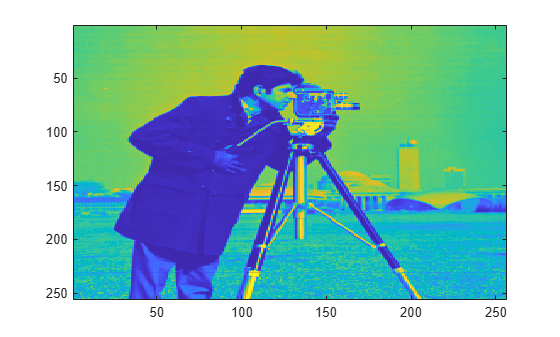# haart2

2-D Haar wavelet transform

## Syntax

``````[a,h,v,d] = haart2(x)``````
``````[a,h,v,d] = haart2(x,level)``````
``````[a,h,v,d] = haart2(___,integerflag)``````

## Description

example

``````[a,h,v,d] = haart2(x)``` performs the 2-D Haar discrete wavelet transform (DWT) of the matrix, `x`. `haart2` returns the approximation coefficients, `a`, at the coarsest level. `haart2` also returns cell arrays of matrices containing the horizontal, vertical, and diagonal detail coefficients by level. If the 2-D Haar transform is computed only at one level coarser in resolution, then `h`, `v`, and `d` are matrices. The default `level` depends on the number of rows of `x`.```

example

``````[a,h,v,d] = haart2(x,level)``` performs the 2-D Haar transform down to the specified level. ```

example

``````[a,h,v,d] = haart2(___,integerflag)``` specifies how the 2-D Haar transform handles integer-valued data, using any of the previous syntaxes.```

## Examples

collapse all

Obtain the 2-D Haar transform of 2-D data and plot its diagonal and horizontal level 1 details.

```load xbox; [a,h,v,d] = haart2(xbox); imagesc(xbox) title('Original Image')``````figure subplot(2,1,1) imagesc(d{1}) title('Diagonal Level 1 Details') subplot(2,1,2) imagesc(h{1}) title('Horizontal Level 1 Details')```Show the effect of limiting the maximum level of the 2-D Haar transform on an image.

Load and display the image of a cameraman.

```im = imread('cameraman.tif'); imagesc(im)```Obtain the 2-D Haar transform to level 2 and view the level 2 approximation.

```[a2,h2,v2,d2] = haart2(im,2); imagesc(a2)```Compare 2-D Haar transform results using the default `'noninteger'` flag and the `'integer'` flag. The cameraman image is `uint8` data, so its maximum value is 255.

Obtain the default Haar transform. The approximation detail coefficient is outside the range 0 to 255.

```im = imread('cameraman.tif'); [a,h,v,d] = haart2(im); a```
```a = 3.0393e+04 ```

Obtain the Haar transform, limiting it to integer values. The approximation detail is an integer and is within the range of the original image data.

```[a,h,v,d] = haart2(im,'integer'); a```
```a = 119 ```

## Input Arguments

collapse all

Input signal, specified as a 2-D or 3-D matrix of real values. If `x` is 3-D, the third dimension of `x` must equal 3. The row and column sizes of `x` must be even length.

Data Types: `double`

Maximum level to which to perform the 2-D Haar transform, specified as a positive integer. The default value depends on the length of the input signal, `x`.

• If both the row and column sizes of `x` are powers of two, the 2-D Haar transform is obtained down to `level` `log2(min(size(x)))`.

• If both the row and column sizes of `x` are even, but at least one is not a power of two, `level` is equal to `floor(log2(min(size(x)/2)))`.

If `level` is greater than 1, then `h`, `v`, and `d` are cell arrays. If `level` is equal to 1, then `h`, `v`, and `d` are matrices.

Integer-valued data handling, specified as either `'noninteger'` or `'integer'`. `'noninteger'` does not preserve integer-valued data in the 2-D Haar transform, and `'integer'` preserves it. The `'integer'` option applies only if all elements of the input, `x`, are integers. For integer-valued input, `haart2` returns integer-valued wavelet coefficients. For both `'noninteger'` and `'integer'`, however, the 2-D Haar transform algorithm uses floating-point arithmetic. The data type of outputs `a`, `h`, `v`, and `d`, is always `double`.

## Output Arguments

collapse all

Approximation coefficients, returned as a scalar or matrix of coefficients, depending on the level to which the transform is calculated. Approximation, or scaling, coefficients are a lowpass representation of the input. At each level, the approximation coefficients are divided into coarser approximation and detail coefficients.

Data Types: `double`

Horizontal detail coefficients by level, returned as a matrix or cell array of matrices. If `level` is greater than 1, `h` is a cell array. If `level` is equal to 1, the 2-D Haar transform is computed at only one level coarser in resolution and `h` is a matrix.

Note: Generated C and C++ code always returns the horizontal detail coefficients `h` in a cell array.

Data Types: `double`

Vertical detail coefficients by level, returned as a matrix or cell array of matrices. If `level` is greater than 1, `v` is a cell array. If `level` is equal to 1, the 2-D Haar transform is computed at only one level coarser in resolution and `v` is a matrix.

Note: Generated C and C++ code always returns the vertical detail coefficients `v` in a cell array.

Data Types: `double`

Diagonal detail coefficients by level, returned as a matrix or cell array of matrices. If `level` is greater than 1, `d` is a cell array. If `level` is equal to 1, the 2-D Haar transform is computed at only one level coarser in resolution and `d` is a matrix.

Note: Generated C and C++ code always returns the diagonal detail coefficients `d` in a cell array.

Data Types: `double`

## Extended Capabilities

### C/C++ Code GenerationGenerate C and C++ code using MATLAB® Coder™.